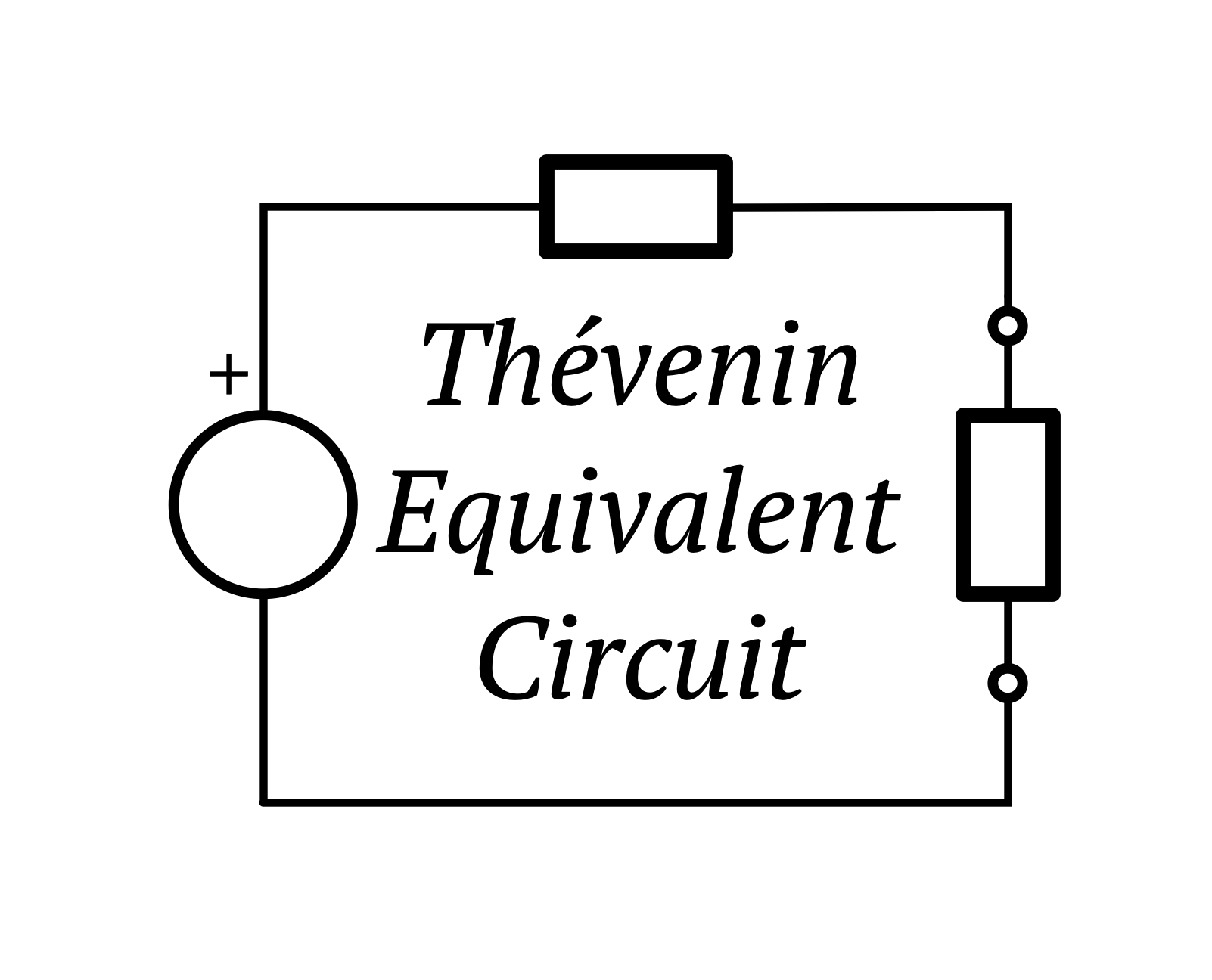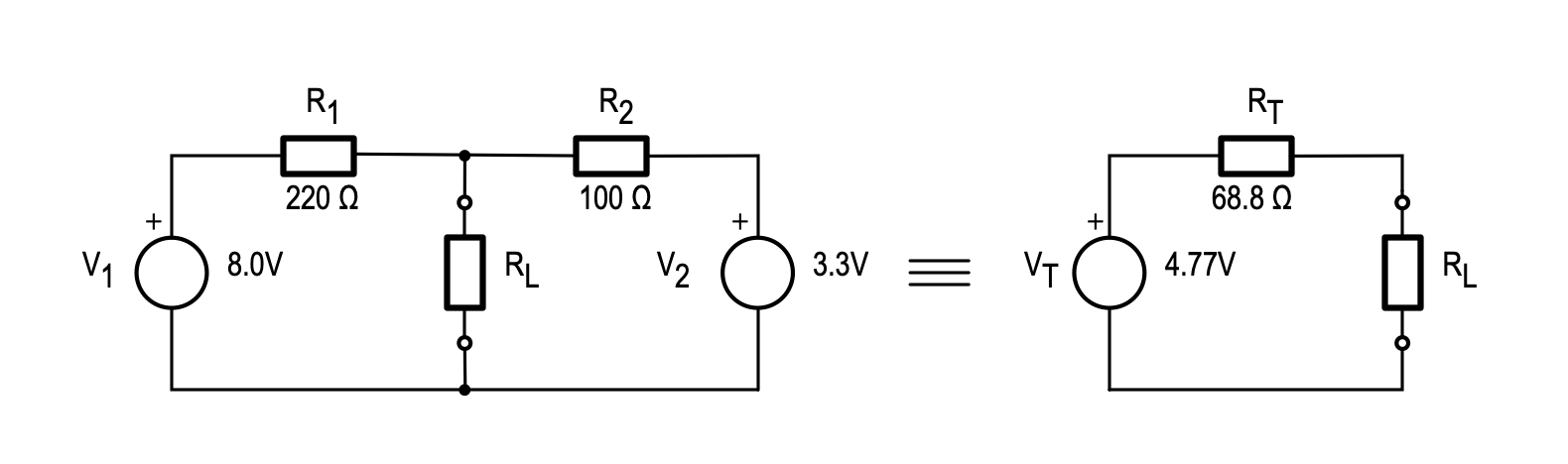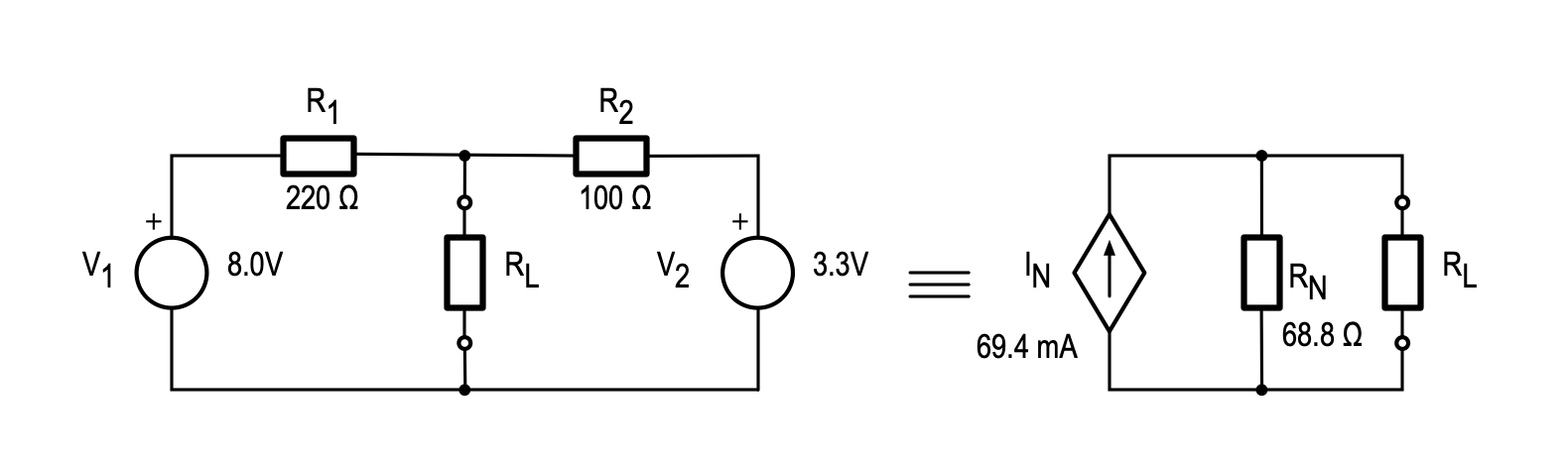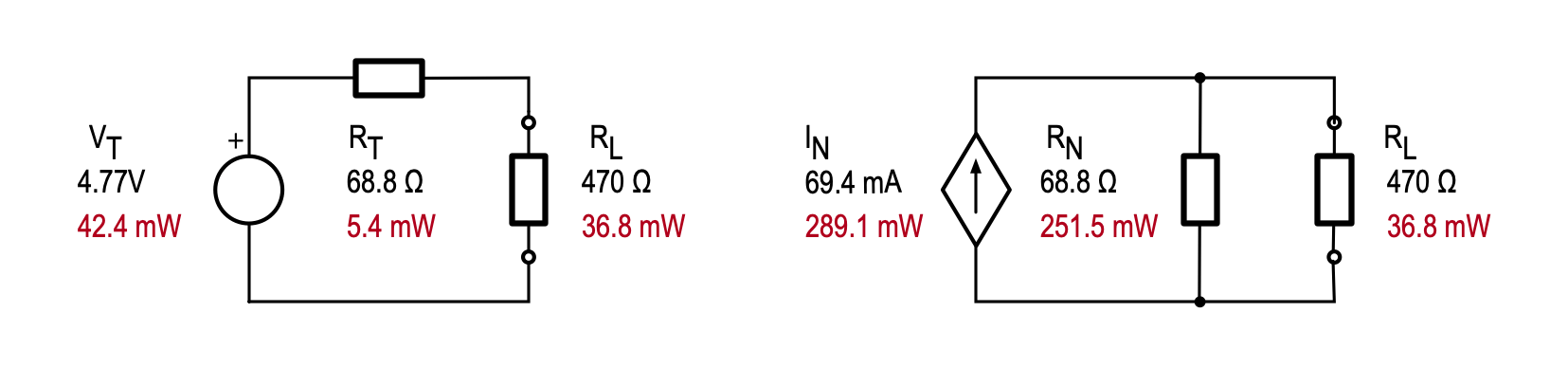# Thévenin & Norton Equivalent CircuitsWritten by

Back in 1882, a French engineer, Léon Charles Thévenin, got interested in circuit theory. Building on Ohms’ law and Kirchoff’s law he developed a (then controversial) idea that any circuit with any combination of resistances, voltage and current sources could be reduced to an equivalent circuit with just one voltage source and one resistor.

This theorem is very useful in the analysis of circuits where there are multiple power sources, such as that shown on the left side of Figure 1. Here we have a load resistor, RL being fed by two voltage sources V1 add V2, each via a resistance R1 and R2 respectively. If we are interested in the behavior of this circuit with various load conditions, it would be much easier if we could reduce these to a single voltage source, VT and series resistor, RT as shown on the right side of Figure 1. Thévenin says we can do this and shows us how.FIGURE 1. The Thévenin equivalency theorem says that we can define a circuit with a single voltage source VT and a single series resistor RT (at right) that has identical behavior as far as the load is concerned, for any combination of sources and resistances such as the example circuit on the left.

First let’s imagine the load RL, is removed as shown in Figure 2.FIGURE 2. We can determine VT by calculating (or measuring) the terminal voltage with no load (top). We can determine RT by setting the sources to zero and calculating (or measuring) the resistance seen between the terminals.

The output voltage we see at the terminals must be VT if the circuits are equivalent. We can see that this is V1 less the voltage dropped across R1:

Using the values shown, we get a value for VT of 4.77V. To calculate the Thévenin equivalent resistance RT, we can set the voltage sources to zero and look at the resistance seen at the terminals. This will obviously be the parallel combination of R1 and R2 which is 68.8 Ω in our example. The Thévenin equivalent circuit is therefore that of Figure 3. This equivalency can be applied to a circuit with any combination of sources (including current sources) and resistances regardless of the complexity.FIGURE 3. The Thévenin equivalent circuit (on the right) looks identical to the original circuit (on the left) as far as the load is concerned.

In one of those coincidences that seem to be common in science and technology, in 1926, both Hans Ferdinand Mayer and Edward Lawry Norton, each independently discovered a second equivalency, in which any circuit with a Thévenin equivalent can also be described by a single current source in parallel with a single resistor. For some reason, this equivalent is universally known as a Norton equivalent circuit. Herr Mayer seems to have been entirely forgotten, which is sad since he has a very interesting life story outside of engineering.

To find the Norton equivalent circuit we go through a similar process as we did before. First, we find the Norton equivalent current (IN) by shorting out the load terminals as shown in Figure 4.FIGURE 4. We can determine the Norton equivalent current IN by calculating (or measuring) the current with the terminals shorted (top). We can determine RN by setting the sources to zero and calculating (or measuring) the resistance seen between the terminals.

The current that flows through the short must be equal to IN, and is the sum of the currents through R1 and R2:

In our example this will be 69.4mA. The Norton equivalent resistance (RN) is similarly determined by looking into the terminals with the source set to zero. This will be the same as for the Thévenin case since an ideal current source has infinite resistance. The resulting Norton equivalent circuit is shown in Figure 5.FIGURE 5. The Norton equivalent circuit (on the right) looks identical to the original circuit (on the left) as far as the load is concerned.

One really important point to note is that these circuits are only equivalent from the perspective of the load. The voltage and current through the load will be the same under all circumstances, whether connected to the original circuit, or either of the equivalents. The equivalent circuits themselves are not identical in behavior. Figure 6 shows the power dissipation in both equivalent circuits when a 470Ω load is applied. In each case the power dissipated in the load is 36.8mW, but the Thévenin equivalent resistor dissipates 5.4mW whereas the Norton equivalent resistance dissipates 252 mW—clearly these circuits not the same as each other.FIGURE 6. While these circuits are equivalent as far as the load is concerned – the voltage, current and power are the same for any given load – these circuits themselves do not behave equivalently. For example, the power dissipated in RT and RN are very different, despite them having the same value. Similarly, the power delivered by the two sources is different.

References:

“Hans Ferdinand Mayer.” In Wikipedia, August 25, 2020. https://en.wikipedia.org/w/index.php?title=Hans_Ferdinand_Mayer&oldid=974870246.

“Edward Lawry Norton.” In Wikipedia, March 10, 2021. https://en.wikipedia.org/w/index.php?title=Edward_Lawry_Norton&oldid=1011318764.

“Léon Charles Thévenin.” In Wikipedia, February 14, 2021. https://en.wikipedia.org/w/index.php?title=L%C3%A9on_Charles_Th%C3%A9venin&oldid=1006674298.

 Keep up-to-date with our FREE Weekly Newsletter! Don't miss out on upcoming issues of Circuit Cellar.— ADVERTISMENT— —Advertise Here— Subscribe to Circuit Cellar Magazine Note: We’ve made the May 2020 issue of Circuit Cellar available as a free sample issue. In it, you’ll find a rich variety of the kinds of articles and information that exemplify a typical issue of the current magazine. Would you like to write for Circuit Cellar? We are always accepting articles/posts from the technical community. Get in touch with us and let's discuss your ideas.